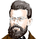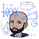TradingViewצפיות ‎8365‎
This is an updated version of my "Black-Scholes Model and Greeks for European Options" indicator, that i previously published. I decided to make this updated version open-source, so people can tweak and improve it.

The Black-Scholes model is a mathematical model used for pricing options. From this model you can derive the theoretical fair value of an options contract. Additionally, you can derive various risk parameters called Greeks. This indicator includes three types of data: Theoretical Option Price (blue), the Greeks (green), and implied volatility (red); their values are presented in that order.

1) Theoretical Option Price:
This first value gives only the theoretical fair value of an option with a given strike based on the Black-Scholes framework. Remember this is a model and does not reflect actual option prices, just the theoretical price based on the Black-Scholes model and its parameters and assumptions.

2)Greeks (all of the Greeks included in this indicator are listed below):

a)Delta is the rate of change of the theoretical option price with respect to the change in the underlying's price. This can also be used to approximate the probability of your option expiring in the money. For example, if you have an option with a delta of 0.62, then it has about a 62% chance of expiring in-the-money. This number runs from 0 to 1 for Calls, and 0 to -1 for Puts.

b)Gamma is the rate of change of delta with respect to the change in the underlying's price.

c)Theta, aka "time decay", is the rate of change in the theoretical option price with respect to the change in time. Theta tells you how much an option will lose its value day by day.

d) Vega is the rate of change in the theoretical option price with respect to change in implied volatility .

e)Rho is the rate of change in the theoretical option price with respect to change in the risk-free rate. Rho is rarely used because it is the parameter that options are least effected by, it is more useful for longer term options, like LEAPs.

f)Vanna is the sensitivity of delta to changes in implied volatility . Vanna is useful for checking the effectiveness of delta-hedged and vega-hedged portfolios.

g)Charm, aka "delta decay", is the instantaneous rate of change of delta over time. Charm is useful for monitoring delta-hedged positions.

h)Vomma measures the sensitivity of vega to changes in implied volatility .

i) Veta measures the rate of change in vega with respect to time.

j)Vera measures the rate of change of rho with respect to implied volatility .

k)Speed measures the rate of change in gamma with respect to changes in the underlying's price. Speed can be used when evaluating delta-hedged and gamma hedged portfolios.

l)Zomma measures the rate of change in gamma with respect to changes in implied volatility . Zomma can be used to evaluate the effectiveness of a gamma-hedged portfolio.

m)Color, aka "gamma decay", measures the rate of change of gamma over time. This can also be used to evaluate the effectiveness of a gamma-hedged portfolio.

n)Ultima measures the rate of change in vomma with respect to implied volatility .

o)Probability of Touch, is not a Greek, but a metric that I included, which tells you the probability of price touching your strike price before expiry.

3) Implied Volatility:
This is the market's forecast of future volatility . Implied volatility is directionless, it cannot be used to forecast future direction. All it tells you is the forecast for future volatility .

How to use this indicator:
1st. Input the strike price of your option. If you input a strike that is more than 3 standard deviations away from the current price, the model will return a value of n/a.
2nd. Input the current risk-free rate.(Including this is optional, because the risk-free rate is so small, you can just leave this number at zero.)
3rd. Input the time until expiry. You can enter this in terms of days, hours, and minutes.
4th.Input the chart time frame you are using in terms of minutes. For example if you're using the 1min time frame input 1, 4 hr time frame input 480, daily time frame input 1440, etc.
5th. Pick what style of option you want data for, European Vanilla or Binary.
6th . Pick what type of option you want data for, Long Call or Long Put.
7th . Finally, pick which Greek you want displayed from the drop-down list.

*Remember the Option price presented, and the Greeks presented, are theoretical in nature, and not based upon actual option prices. Also, remember the Black-Scholes model is just a model based upon various parameters, it is not an actual representation of reality, only a theoretical one.

*Note 1. If you choose binary, only data for Long Binary Calls will be presented. All of the Greeks for Long Binary Calls are available, except for rho and vera because they are negligible.

*Note 2. Unlike vanilla european options, the delta of a binary option cannot be used to approximate the probability of the option expiring in-the-money. For binary options, if you want to approximate the probability of the binary option expiring in-the-money, use the price. The price of a binary option can be used to approximate its probability of expiring in-the-money. So if a binary option has a price of \$40, then it has approximately a 40% chance of expiring in-the-money.

*Note 3. As time goes on you will have to update the expiry, this model does not do that automatically. So for example, if you originally have an option with 30 days to expiry, tomorrow you would have to manually update that to 29 days, then the next day manually update the expiry to 28, and so on and so forth .

There are various formulas that you can use to calculate the Greeks. I specifically chose the formulations included in this indicator because the Greeks that it presents are the closest to actual options data. I compared the Greeks given by this indicator to brokerage option data on a variety of asset classes from equity index future options to FX options and more. Because the indicator does not use actual option prices, its Greeks do not match the brokerage data exactly, but are close enough.

I may try to make future updates that include data for Long Binary Puts, American Options, Asian Options, etc.
הערות שחרור: Long Binary Puts are now available.
הערות שחרור: Asian Options are now available.

Asian Options, aka Average Options, are a class of exotic options where instead of needing price to be above a strike price upon expiry (long call) or below a strike (long put), the average price needs to be above (long call) or below (long put) the strike upon expiry. There are a few ways in which to calculate the average; Asian options can be based on the arithmetic average, geometric average, or harmonic average.

Here are the differences between average types:

For example say the prices of the last 3 periods are: 5,2,3.

Arithmetic: (5+2+3)/3

Geometric: (5*2*3)^(1/3)

Harmonic: 3/((1/5)+(1/2)+(1/3))

This model provides theoretical pricing for geometric asian options. Geometric Asian Options can be picked from the drop down menu. If chosen the only greeks that won't work are rho, vera, and probability of touch. Because there aren't any readily available Asian option data to compare with, I don't know how close the greeks for the geometric asian options would match realtime data, and therefore are theoretical in nature.
הערות שחרור: Fixed minor mathematical error in the Cumulative Distribution Function.
הערות שחרור: In this update I fixed the implied volatility calculation, which in turn makes Gamma and Theta more accurate. I also changed the formulation of vega, utilizing a formula that provides a vega value more in line with actual brokerage options data. Additionally, I updated the Binary Vega formula.
הערות שחרור: In this update I completely overhauled the code. Doing some research online, I found some java code that calculated the Black Scholes model and I translated it into pine script. This newer version provides way more accurate options pricing and Greeks.

Additionally, I updated the IV calculation to make it more accurate. Since I am using a calculation that does not involve option prices, the IV values will not be exactly the same as ones provides from external sources, like brokers, exchanges, etc; but are close enough. The only disclaimer is that with the IV generated by this script, I would not recommend using it if your expiry is more than 100 days. For some reason with expiries over 100 days the IV values become way off. This is why with this update you now have the choice of using your own IV values or the ones generated by this script. If you have your own source for IV such as from a broker, exchange, etc you can input it into this model and specify this in the dropdown menu.

Providing your own IV values will generate the most accurate options pricing and Greeks. But if you decide to use the script-generated IV values, the pricing and Greeks should still be close to actual data, with the previously mentioned disclaimer in mind.

Also, as a reminder this script does not auto-update the days until expiry, so you will have to update this yourself as time moves on.

Hope this update helps!
הערות שחרור: Removed some redundant code.
הערות שחרור: This new update allows you to choose the base currency of the options contract. By picking the base currency this will input the risk free rate of the corresponding country. You can choose base currencies in USD, GBP, JPY, CAD, CNH, SGD, INR, AUD, SEK, NOK, and DKK.
הערות שחרור: I want to preface this update by saying that it was recently brought to my attention that the method used to calculate my Black-Scholes model in the previous update was similar to the one used by @racer8. Both the previous version and Racer8's version use an error function to calculate the BS model. Since Racer8 came out with their version utilizing an error function first, I decided to change the way in which mine is calculated. If you are reading this, please check out Racer8's Black Scholes model, which utilizes an error function. Racer8's version is really great and works well. If you decide to like my script, please also check out Racer8's version and give it a like as well.

As for the update, the only major difference is that instead of using an error function to calculate the Black-Scholes model, this update uses probability distribution functions and cumulative distribution functions.
הערות שחרור: Just updated comments and removed extraneous code.
הערות שחרור: Fixed calculation of pi.
הערות שחרור: Optimized pi calculation.
סקריפט קוד פתוח

ברוח TradingView אמיתית, מחבר סקריפט זה פרסם אותו עם קוד פתוח, כך שסוחרים יוכלו להבין ולאמת זאת. הידד למחבר! אתה יכול להשתמש בו בחינם, אך שימוש חוזר בקוד זה בפרסום הנו בכפוף ל כללי הבית . אתה יכול להגדירו כמועדף ולהשתמש בו בגרף.

רוצה להשתמש בסקריפ זה בגרף?

## תגובותI have compiled this code with Python 3.8.2, but it is not passed. Why? please suggest me.
השבSegaRKO
@SegaRKO, Thank you so much, you are great, I am studying the European option, I will ask you soon about this.
השבThis publication is now featured in our Editors' Picks: https://www.tradingview.com/scripts/editors-picks/ .
In the name of all TradingView traders, thank you for your valuable contribution to the TradingView community, and congrats!
השבPineCoders
@PineCoders, some parts are lifted and the cdf is incorrect.
השבThank you for this. Do you know if this can be used on stocks and futures?
השב